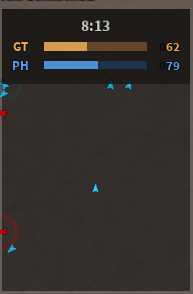# How do i make a radar like this

I want to make a radar like the image below (image from phantom forces):I have got the radar script working but I could not find out a way to make the objects in the radar stay at the frame borders when it goes off the boundaries

How do i do this
(btw this is my first devforum post)

1 Like

I would assume you could use `math.clamp` to set the limits to the calculation of where the players are inside of the radar frame. Obviously you’ll need to adapt this to your script but the logic is still the same.

``````local maxX, maxY = Frame.AbsoluteSize.X, Frame.AbsoluteSize.Y
local minX, minY = 0, 0

local CalculatedX = -- Provide the calculation of the players X position
local CalculatedY = -- Provide the calculation of the players Y position
local NewRadarPosition = UDim2.fromOffset(math.clamp(CalculatedX, minX, maxY), math.clamp(CalculatedY, minY, maxY))``````
2 Likes

My position is currently set up like this (friend helped me set this up):

``````local cameracf = cf(torso.CFrame.p, torso.CFrame.p + look) --torso is actually the humanoid root part of the player
local diff = cameracf:inverse() * tor.Position --tor here is the target's humanoid root part
local x = 0.5 + diff.x / distance --distance is a fixed value, 300 in this case
local z = 0.5 + diff.z / distance
local pos = UDim2.new(x, offset, z, offset) --offset is the size of the dot divided by 2
``````

Since it is already a UDim2, what do i do here?
is it supposed to be something like this?

``````pos = UDim2.new(math.clamp(x, minX, maxY), offset, math.clamp(z, minX, maxY), offset)
``````
1 Like

Since you’re using Scale your `maxX` & `maxY` would be 1 instead of the AbsoluteSize of the frame since that uses offset. I’m unsure how the offset will effect it, let me know how it does when testing.

There are many resources out here which provides that. For example: Radar system for games

This topic was automatically closed 14 days after the last reply. New replies are no longer allowed.